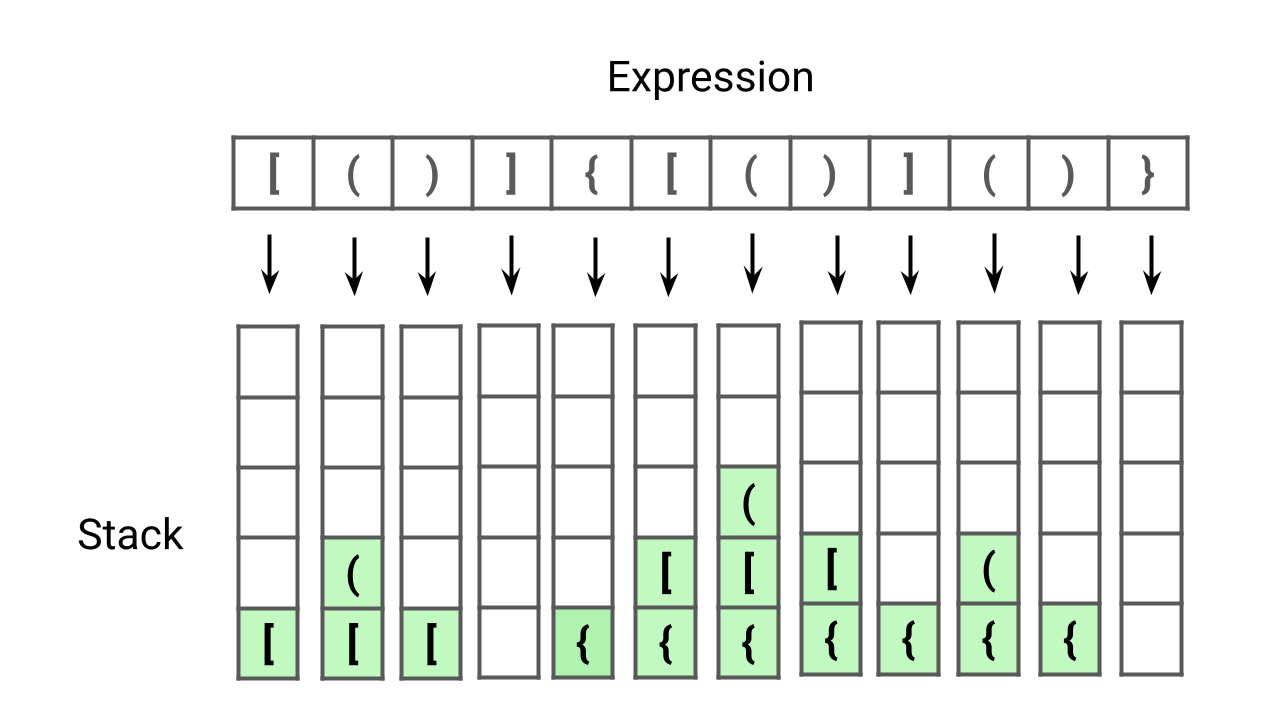# stringValid Parentheses Expression

Given an expression string containing opening and closing parentheses, write a program to check if the expression is balanced or not. An expression is considered balanced if each opening parenthesis is closed by the same type of closing parenthesis in the exact same order. This problem is excellent for learning problem-solving using stacks.Longest Common Subsequence

Given two strings X[] and Y[] of sizes m and n, design an algorithm to find the length of the longest common subsequence (LCS). There can be many possible common subsequences of two strings, but we need to return the common subsequence of the longest length. Note that this is an excellent problem to learn dynamic programming.Longest Substring Without Repeating Characters

Given a string S, write a program to find the length of longest substring without repeating characters. The substring is a continuous subpart of the string and we need to return the largest substring which has all unique characters. Note: This is an excellent problem to learn problem solving and time complexity optimization using sliding window approach.FizzBuzz Problem

Given an integer n, write a program to return string representation of numbers from 1 to n. If number is divisible by 3, we store “Fizz”. If number is divisible by 5, we store “Buzz”. If number is divisible by both 3 and 5, we store “FizzBuzz”. Hint: This is an excellent puzzle to learn coding concepts like conditional statements, loops, etc.Check whether two strings are anagram or not

Given two strings, str1 and str2, of size m and n, write a program to check whether two strings are an anagram of each other or not. A string str1 is an anagram of str2 if characters of str1 can be rearranged to form str2. Note: This is an excellent string problem to learn problem-solving and optimization using direct address table.Roman to Integer

Given a Roman number, write a program to convert Roman number to a corresponding integer value. What are Roman numbers? Roman numbers are the symbols used in a system of numerical notation based on the ancient Roman system. The symbols are I, V, X, L, C, D, and M, which represent integer values respectively for 1, 5, 10, 50, 100, 500, and 1,000.Sort Characters by Frequency

Given a string S[], write a program to sort string S in decreasing order based on the frequency of characters. The frequency of a character is the number of times it appears in the string. If two characters have the same frequency, whichever occurs earliest in S, must come first. In other words, frequency based sorting must be stable.